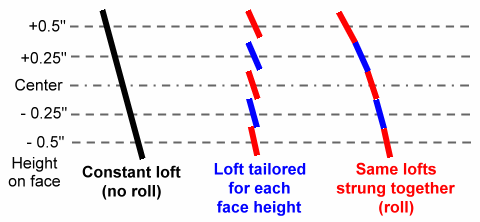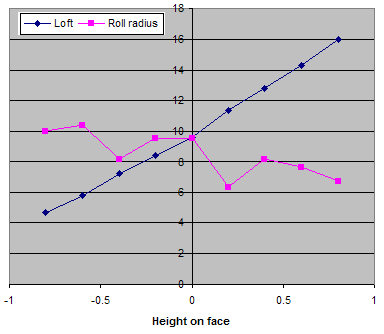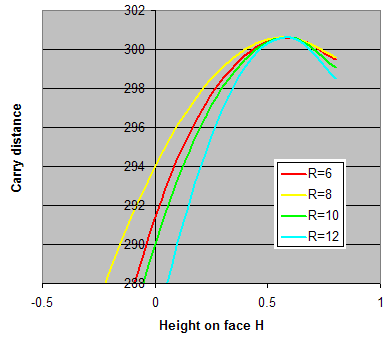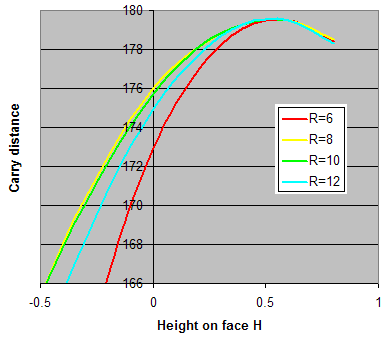All about Gear Effect - 5
Dave Tutelman  --  February 22, 2009

## The role of roll

After the previous pages were posted to my web site, Marcel Bal asked me about face roll. Specifically, he wanted to know if vertical face curvature was a good thing or a bad thing. He mentioned Tom Wishon's GRT (graduated roll technology) drivers, which are specifically designed with minimal face roll except fairly high on the clubface -- and even then then a relatively small roll.

What a wonderful question! And why didn't I think to ask it before I posted the article? It would seem a logical culmination of all that work, because it requires almost everything to give a complete answer. In fact, it probably calls for correcting for clubhead rotation; even though the rotation is small during impact, it can have a non-neglible effect on the answer to this question.

Let's see if we can come up with an answer.

### General approach

We clubmakers tend to think of a single loft number as the ideal loft for a specific swing, where a swing is a combination of clubhead speed and angle of attack, and perhaps other effects like shaft bend and wrist position at impact. I won't debate exactly what goes into it, but rather address the idea that the ideal loft is a single number at all heights on the clubface.

When we look for the ideal loft, we vary the loft while looking at launch conditions -- ball speed, launch angle, and backspin -- and how the combination affects the distance. Our simple model of  ideal loft therefore assumes that, for a given swing, the launch conditions (especially the backspin) is the same wherever on the clubface you strike the ball. Now we know that assumption is wrong!Figure 5-1

Figure 5-1 shows the clubface angle (the loft) at various heights on the face, specifically at the center and a quarter-inch and half-inch above and below the center. The black line on the left is a constant-loft face (in other words, no curvature, no face roll). Are the launch conditions going to be the same at each height? In a word, no!
• The backspin will vary quite a lot. The data we used to validate vertical gear effect showed a variation of 1300rpm over this same range of heights.
• The ball speed and launch angle may vary a small amount, because of face rotation caused by a high or low impact.
So we can't just assume that the same loft is the ideal, or optimum, loft at each height on the face. The red and blue segments are a more realistic picture. We can look at the launch conditions at each face height and optimize the loft for that height, taking into account face rotation (and thus gear effect). We already know that the backspin will be less as we move up the face. A little work with any trajectory program tells us emphatically that decreased backspin calls for increased launch angle, and vice versa. So we can expect that high on the clubface (low backspin) the best loft should be higher than the center of the face.  Conversely, the backspin is higher at the bottom of the face, so we can expect the optimum loft there to be lower.

If we take these ideal loft segments and string them together, as we do at the right on Figure 5-1, we see that the progression of lofts requires a curvature of the clubface. So, if we have a collection of ideal lofts, that implies what the ideal face roll should be.
How can we go from a set of optimum lofts at various face heights to roll radius? Actually, that's the simple part. (The hard work was finding the optimum loft at each face height.)Figure 5-2

Figure 5-2 shows a driver head, with the loft specified at two points. The two lofts are L1 and L2, and they are separated by a height difference of ΔH. What we will do is find a series of lofts L1, L2, etc, at height increments of ΔH.

Those lofts constitute a curvature statement. But we are used to seeing the curvature in terms of radius -- as roll is usually specified. We will have to turn that series of lofts into a series of radii of curvature. If they are all pretty similar, we can use the average as a fair description of the roll radius for the whole clubface. If there is a lot of variation, then the ideal clubface has a graduated roll. This may be the same as Wishon's GRT graduated roll -- or different. We're going to find out which.

The figure hints at the computation, but let's be explicit. We have drawn two radii, perpendicular to the face at the two points of interest. Where they meet is the radius of curvature, at least for the section of clubface between the two points. The angle at which they meet is the difference between the lofts
ΔL  = L2 - L1
The angle ΔL as a proportion of 360° is the same as ΔH as a proportion of the circumference 2πR, or:
 ΔL 360° = ΔH 2πR
Solving the proportion for R, we get:
 R  = 360 2π ΔH ΔL =  57.3 ΔH ΔL

So the steps are:
1. Choose a set of heights on the clubface. We will choose -0.8" to +0.8" by 0.2" increments.
2. Optimize the loft at each point.
3. Look at the optimized lofts, and draw some conclusions about face roll.
Let's do it!

### Equations we will need

If you're interested in the results but not the math, skip this sub-chapter.

We already have, or can easily find from what we've already done, the equations we need. They are summarized in the table below.

What it finds Formula Where or how
we got it
Ball speed  Vb (mph)
 Vc 1 + e 1 + m/M cos (L)

= 0.813 Vc (1+e) cos (L)
Golf physics
tutorial
Backspin from loft  so  (rpm) 160 Vc sin (L) Golf physics
tutorial
Launch angle  a  (degrees) L (0.96 - .0071 L) Golf physics
tutorial
Torque moment arm  y  (inches) H - D sin a This article,
vertical gear effect
Correction to reduce ball speed
 98 Vb y2 Iv
This is half the
backward velocity
of the face

at release.
See appendix
Correction to increase launch angle
 32.7 Vb y Iv
This is half the
rotation during
impact.
See appendix
Gear effect topspin  s  (rpm) 25 Vb y Basic vertical
gear effect spin

Now we have enough to create a spreadsheet to give us launch conditions for any loft and clubhead speed. We will build the spreadsheet using the above formulae, and then iteratively solve for the optimum loft. That is:
• We will start with a constant clubhead speed (102.7mph, to give 150mph ball speed) and constant loft across the face (11°). Further assumptions about the driver:
• We will assume a constant COR across the face at 0.83, the rules-allowed maximum. Designers have learned how to shape the face thickness to preserve COR across a lot of the face, so this analysis assumes such a driver.
• We continue (as in all the previous examples) with a zero angle of attack.
• For each height on the clubface (H= -0.8" through H= +0.8"), we will do the following:
1. Use the spreadsheet to compute a set of launch conditions (ball speed, launch angle, and backspin).
2. Plug the launch conditions into TrajectoWare Drive (TWD) and find the carry distance and angle of descent.
3. If this is the maximum distance we are likely to get, note it and the loft -- then move on to the next H. Otherwise,  choose a new loft (higher or lower) and go back to step 1.

### Optimized roll results

After we go through those calculations, we will have found the optimum loft for each height on the face. Then we will use that to picture the face roll curvature. And here is the result of those computations.

 Inputs Computed intermediate results Backspin Outputs H Loft Ball speed Launch angle y Correct'd ball speed Correct'd launch angle Due to loft Due to gear effect Net Carry Distance (TWD) Angle of Descent (TWD) Roll radius R -0.8 4.7 152.2 4.4 -0.90 148.3 2.9 1346 3331 4677 213.9 31.9 --- -0.6 5.8 152.0 5.3 -0.72 149.4 4.2 1660 2692 4353 221.7 31.8 10.4 -0.4 7.2 151.6 6.5 -0.55 150.1 5.7 2059 2057 4117 228.5 34.0 8.2 -0.2 8.4 151.2 7.6 -0.37 150.5 7.0 2400 1396 3797 233.9 34.2 9.6 0 9.6 150.7 8.6 -0.19 150.5 8.3 2740 728 3469 237.9 34.1 9.6 0.2 11.4 149.8 10.0 -0.03 149.8 10.0 3247 99 3347 241.0 35.4 6.4 0.4 12.8 149.0 11.1 0.15 148.9 11.4 3640 -553 3087 242.6 36.8 8.8 0.6 14.3 148.1 12.3 0.32 147.6 12.8 4058 -1192 2867 242.8 37.4 7.6 0.8 16 146.9 13.6 0.50 145.7 14.3 4529 -1803 2726 242 38.6 6.7Figure 5-3 When we look at the main result, the roll radius at each height on the face, we see neither a constant number nor a smooth curve. There is a trend, but it is a jagged one, as shown in the graph at the right. It looks like the optimum face roll is in the 8"-10" range on the lower part of the face, and the 6"-8" range on the upper part. Why should it be this "noisy" a relationship? Take a look at the formula for roll radius. It is proportional to ΔH / ΔL, which is the slope of the height vs loft curve. But we are restricting our search to the best loft to a tenth of a degree. That restricts ΔL to the nearest tenth of a degree, which limits the computed roll to a few discrete values, not a continuum. That is the major reason for "noise". By way of further explanation, the distance vs loft curve is fairly flat near its optimum -- and we are working near the optimum. That means that we could be off by a tenth of a degree of loft. As we saw, numerical differentiation amplifies error. A tenth of a degree error in two successive readings could throw off the face roll by 2 inches. In fact, even the trend might be wrong; the correct answer might be a constant roll or, for that matter, a bigger difference between the top and bottom of the clubface. Why? The lower quarter of the clubface (H≤ -0.4) shows very high net spin -- over 4000rpm -- because the loft spin is adding to a strong gear effect backspin. But TrajectoWare Drive starts to lose accuracy at spins over 4000rpm. So we cannot take very seriously any results from the bottom of the clubface. Finally, it is worth remembering that we assume a 0.83 COR across the entire height of the clubface. Any "sweet spot" effect is due entirely to roll radius and gear effect. If the face is hotter in some spots than others, the COR profile has to be superimposed on our work, and may affect the optimum loft at some of the points. Even with all these caveats, there is a reasonable conclusion to be drawn from the data. The face that the best possible loft progresses with height on the face tells us that the best possible face must be curved. Thus... Face roll is useful in keeping the distance up for high and low mishits. How useful? Let's run our spreadsheet a few more times and see.

### How sensitive is distance to the roll radius?

This time, instead of finding the loft (L) at each face height (H), we will assume a roll radius (R) and compute the distance at each face height. The loft at each height is easy enough to compute, knowing the roll radius. Remember the equation we used earlier:
R  =  57.3 ΔH / ΔL
If we choose some base Ho and Lo for the clubhead, we can find the loft at any face height by solving that equation.
 L  =  Lo + 57.3 H - Ho R
We will continue to use a clubhead speed of 102.7mph, giving a center-hit ball speed of 150mph. From our previous work, we know that the maximum carry distance occurs at about H=½" or perhaps a fraction higher, and the best loft at H=½" is 13.6°. Let is use these as Ho and Lo. That way, we have the same maximum distance no matter what the roll radius.Figure 5-4: Ball speed = 150mph
Figure 5-4 is a graph showing how carry distance varies with H for different values of the roll radius R. The graph shows several things pretty clearly:
• A radius of 8" (the yellow curve) seems to give the best preservation of distance as the height of impact varies. It makes for the most forgiving clubhead -- at least forgiving of height errors.
• For R=8", the distance is within a yard of the maximum anywhere from ¼" to ¾" above the center of the face.
• Things are still quite good with a roll radius of 10" (the green curve). We still lose only a yard from ¼" to ¾" above the center. A lower strike, say on-center, is a yard shorter than the 8" roll -- not bad, but probably measurable.
• We see a little more loss of distance at 6" and 12". Now we are losing three yards from a center hit.
• A nearly flat face without roll (R=40") drops off quite severely when struck either high or low of the optimum.

This tells us that roll matters! It is a good thing. It "makes the sweet spot bigger".

A flat face is way too sensitive to the height at which the ball is struck. Yes, it may preserve a nice-looking trajectory; a low strike will not produce a hot worm-burner. But, given the extra backspin due to gear effect with a low strike, the worm-burner needs that spin and will give more distance -- and the "nice trajectory" from the flat face will balloon and fall short.

But is an 8" roll radius really best? It may well be. Then again, we don't see that much curvature on the clubheads that are being sold.  Perhaps the gear effect model may be a little off. The physics is definitely sound, but our estimate of things like vertical moment of inertia may be a little off. Certainly not by an order of magnitude. Most likely not even by a factor of two. But it might be off enough so that the ideal radius is more like 10" or even 12". I would be surprised if it is off by more than that. And anything approaching a flat face has got to be wrong.

You might ask about another possible source of error in our conclusion. TrajectoWare Drive, the software that computed the distance, loses accuracy at higher spin rates. Is that an issue here? No, it is not. Spin rates high enough to give inaccurate distances did show up, but only for distances under 230 yards, lower than the bottom of the graph. None of the data shown on the graph is affected by TrajectoWare Drive error.

### One roll fits all?

 Is 8" -- or, for that matter, is any roll radius -- the best for all golfers? We haven't addressed that issue at all so far. Let's test the one-size-fits-all assertion by trying higher and lower clubhead speeds and seeing how the ideal roll varies. So far, we have assumed in every case that the golfer has a 102.7mph clubhead speed, generating a ball speed of 150mph. Now we'll try ball speeds of 180mph and 120mph.Figure 5-5: Ball speed = 180mph First we'll try a higher clubhead speed. Figure 5-5 is based on a clubhead speed of 122.5mph, which gives a maximum ball speed of 180mph. This would be a pretty big hitter on the PGA Tour, but not the top ten and definitely not a long drive competitor. For this clubhead speed, the best distance occurs at H=0.6" and a loft at that height of 12.5°. The 8" roll radius is still the best. Looking at the second best, this time it is the 6" roll. (At 150mph it was the 10" roll.) So perhaps the ideal roll is a little more curved for higher ball speeds -- a bit above 8" at 150mph and a bit below at 180mph. But it is not a very big difference, considering that 20mph is a huge difference in clubhead speed, and 30mph a huge difference in ball speed.Figure 5-6: Ball speed = 120mph How about lower ball speeds? Let's try a clubhead speed of 83mph, giving a ball speed of 120mph. All the drivers modeled had a 17.2° loft at a point 0.6" above the center of the face. We see the result in Figure 5-6. This time, the 8" roll shares top billing with the 10" roll, suggesting that perhaps a 9" roll might even be a little better. Still, that is not all that different from the higher clubhead speeds.

### Conclusion

Face roll makes a significant difference in how forgiving a driver is to high or low misses. A flat face has a very small "sweet spot" in terms of height; the carry distance falls off sharply if impact is above or below this height.

The optimum face roll varies a bit with clubhead speed and ball speed. But not much. I would estimate the best face roll for a 180mph ball speed is less than 2" different from that for 120mph ball speed. This might be worth working the problem for a long drive competitor, but probably not for someone playing competitive golf. If the roll is optimized for 150mph, the lost distance is less than a yard for any ball speed between 120mph and 180mph (compared with a roll optimized for that ball speed), for any strike at the middle of the face or higher.

The calculations show an optimum face roll in the vicinity of 8". This may or may not be correct, depending on the accuracy of the model and the assumptions involved.

This invites a discussion of sensitivities of the conclusion. The main sensitivity is the actual amount of ball spin due to vertical gear effect. For the range of interest, more gear effect spin requires more face curvature. In particular:
• If the clubhead moment of inertia is higher than the model estimates, then the optimum roll radius may be more (a flatter face).
• If the CG is farther back in the head, then the optimum roll radius may be less (a more curved face).
• If the effect on launch angle and ball speed due to face rotation is higher than we used, then the optimum roll radius may be more (a flatter face).
• If the shaft tip is actually stiff enough to significantly limit vertical gear effect, then the optimum roll radius may be more (a flatter face).
We noted earlier that the model disagrees with two reports of the amount of gear effect spin, Wishon and Upshaw, which certainly do not agree with each other in any event. The model predicts spins much higher than Wishon reports, and closer to Upshaw's. But I was unable to duplicate analytically Upshaw's reported dependency on shaft tip stiffness. Looking at the sensitivites above:
1. If Wishon's much smaller estimate of gear effect spin is correct, then the optimum roll radius would be much greater (a much flatter face). This explains his GRT driver heads, with a 15" roll for the top third and a 20" roll for the bottom two thirds.
2. If Upshaw's shaft-sensitivity of gear effect is correct, then the optimum roll radius would become heavily dependent on the shaft. It might be anywhere from 8" to nearly flat, depending on the shaft used.
Barring either of these extremes, the bullet-points above are unlikely to cause even a factor of two difference in the optimum roll. In real terms, the optimum roll is almost certainly between 8" and 12". For drivers! Hybrids or even fairway woods might be quite different, because their MOI and depth of CG are quite different.